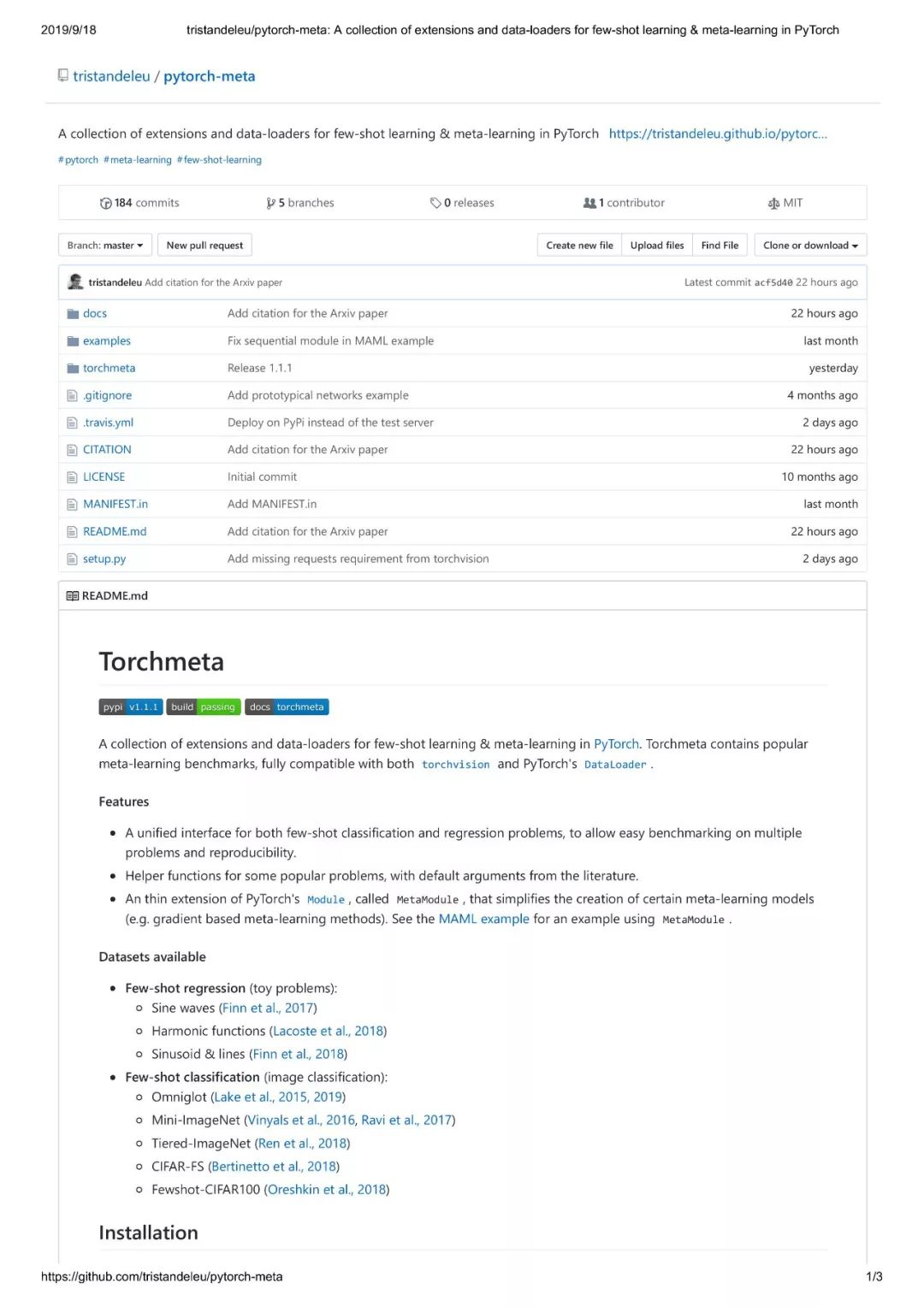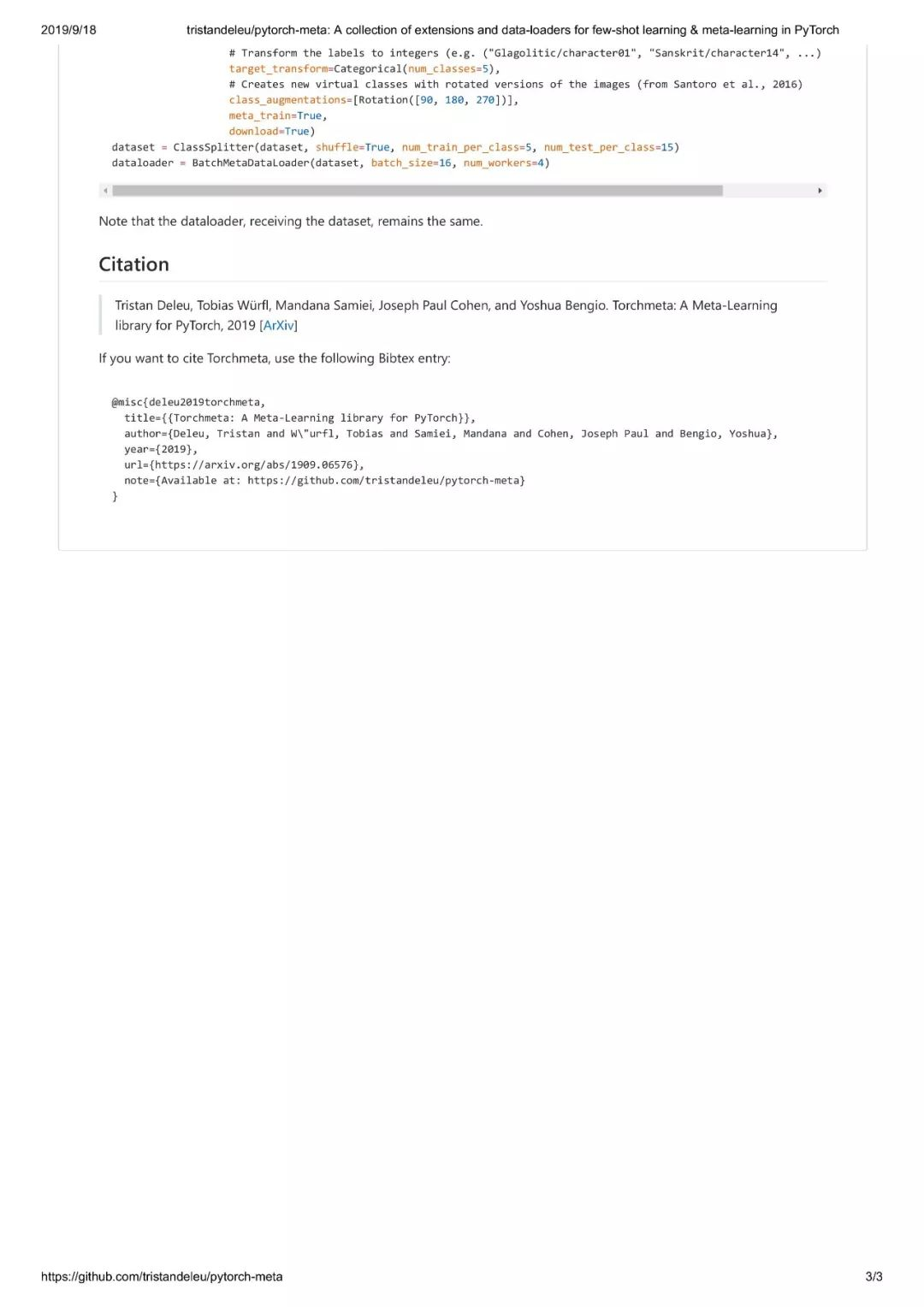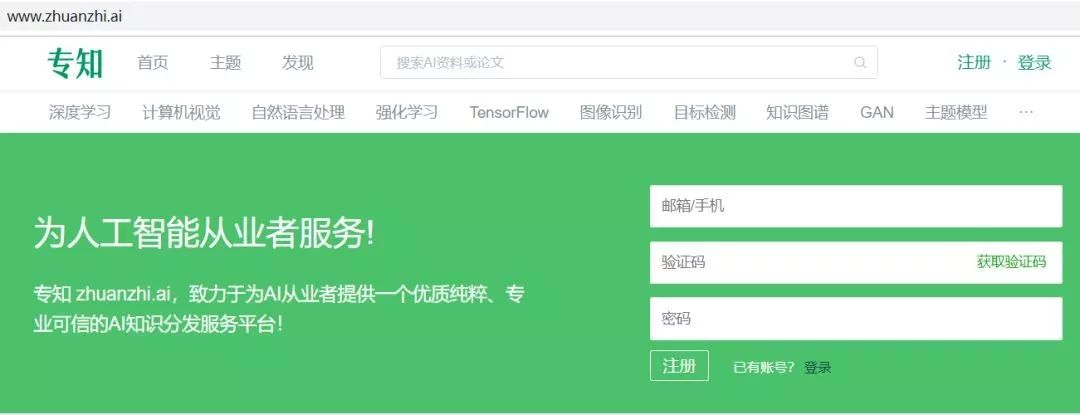## 【资源】PyTorch上方便好用的元学习工具包

9 月 18 日 专知

【导读】元学习是最新的研究热点，也是很多科研人员的跟进方向，本文为大家推荐一个针对PyTorch实现的元学习工具包，方便大家快速检验最先研究想法。

https://github.com/tristandeleu/pytorch-meta-END-0+8+

### 相关内容0+9+0+0+9+0+1+1+0+0+4+0+0+16+0+0+4+0+0+7+0+0+7+0+0+7+0+1+7+0+
Top